# Erratum to "Fixed Points of Maps of a Nonaspherical Wedge"

The Original Article was published on 19 January 2009

## Abstract

In the original paper, it was assumed that a selfmap of, the wedge of a real projective spaceand a circle, is homotopic to a map that takesto itself. An example is presented of a selfmap of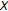that fails to have this property. However, all the results of the paper are correct for maps of the pair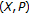.

Let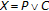be the wedge of the real projective planeand the circle. As the example below demonstrates, the statement on page 3 of  "Given a map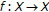we may deformby a homotopy so that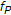, its restriction to, mapsto itself." is incorrect. If, instead of an arbitrary self-map of, we consider a map of pairs, the map can be put in the standard form defined on that page and then all the results of the paper are correct for such maps of pairs.

To describe the example, represent pointsof the unit-sphere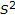by spherical coordinates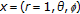wheredenotes the radius,the elevation andthe azimuth. Let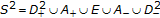whereis in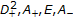or, if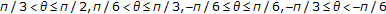or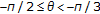, respectively. Let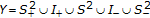, where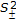are the-spheres of radius one in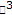with centers, in cartesian coordinates, atdenotes the points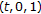for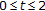and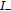the points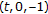for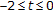. Define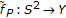in the following manner. For, let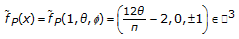(1)

in cartesian coordinates. For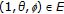, set. Let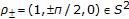be the poles and define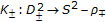by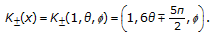(2)

Returning to cartesian coordinates, define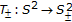by(3)

We complete the definition ofby setting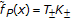for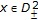. Note that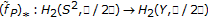such that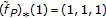. We may embedin the universal covering space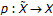because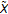is an infinite tree with a 2-sphere replacing each vertex in such a way that two edges are attached at each of two antipodal points. The embedding induces a monomorphism of homology. The map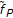has been defined so that ifare antipodal points of, thenand thereforeinduces a map. Ifwere homotopic to a map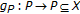, then the homotopy would lift to cover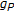by a map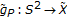which sendsto a single-sphere in. Therefore the image of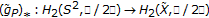would be either trivial or a single generator of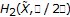. On the other hand, the image of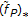inis nontrivial for three generators, so no such homotopy can exist. Therefore, if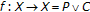is a map whose restriction tois the mapdefined above, then it cannot be homotoped to a map that takesto itself.

## References

1. Kim SW, Brown RF, Ericksen A, Khamsemanan N, Merrill K: Fixed points of maps of a nonaspherical wedge. Fixed Point Theory and Applications 2009, 2099:-18.

## Acknowledgment

The authors thank Francis Bonahon and Geoffrey Mess for their help with the example.

## Author information

Authors

### Corresponding author

Correspondence to RobertF Brown.

The online version of the original article can be found at 10.1155/2009/531037

## Rights and permissions

Reprints and Permissions

Kim, S., Brown, R., Ericksen, A. et al. Erratum to "Fixed Points of Maps of a Nonaspherical Wedge". Fixed Point Theory Appl 2010, 820265 (2010). https://doi.org/10.1155/2010/820265